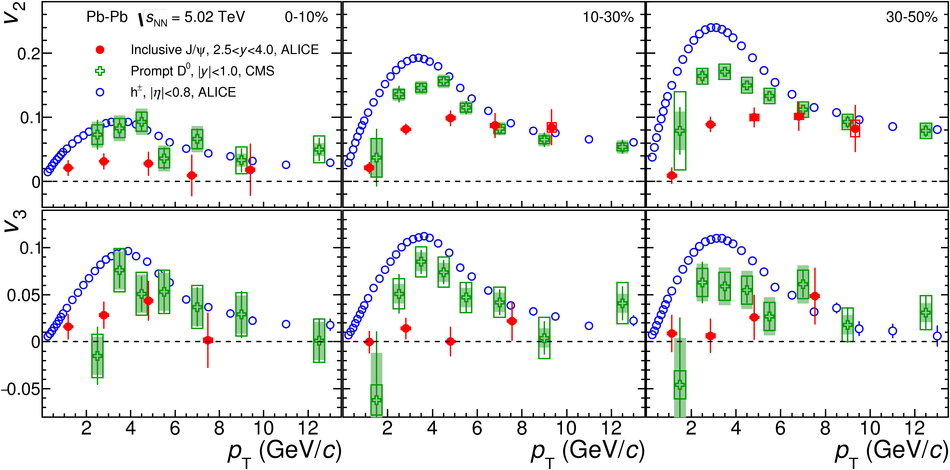# Figure 4

 The \jpsi\ $v_2$ (upper panels) and $v_3$ (bottom panels) coefficients as a function of $p_{\rm T}$ in three centrality intervals (from left to right) in Pb--Pb collisions at $\sqrt{s_{\rm NN}}$ = 5.02 TeV. The results are compared to the $v_2$ and $v_3$ coefficients of mid-rapidity charged particles~ and prompt D$^0$ mesons~. Statistical and systematic uncertainties are shown as bars and boxes, respectively. The shaded bands represent the systematic uncertainties from the contribution of non-prompt D$^0$ mesons.